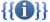# قالب:Order of magnitudeTemplate documentation[view]  [history] [purge]

## فهرست

### Usage

This template calculates the (decimal) order of magnitude of numbers within the ranges 10300 to 10−300 and −10−300 to −10300.

The acceptable input and the output produced are optimised for use in other templates.

• The hyphen (not a true minus sign) is used for negative numbers.
• No separator is used for thousands.
• The template gives 0 for 0. Although, strictly speaking, this is mathematically incorrect it has been designed this way so as to be more useful in other templates. An important example of this is that when used by {{convert}} 0 °C or 0 °F is considered to be on the same order of magnitude as ±1 °C or ±1 °F respectively.

### Examples

• `{{Order of magnitude|123400}}` → 5 
• `{{Order of magnitude|12340}}` → 4 
• `{{Order of magnitude|1234}}` → 3 
• `{{Order of magnitude|123.4}}` → 2 
• `{{Order of magnitude|12.34}}` → 1 
• `{{Order of magnitude|1.234}}` → 0 
• `{{Order of magnitude|.1234}}` → -1 
• `{{Order of magnitude|.01234}}` → -2 
• `{{Order of magnitude|0}}` → 0 
• `{{Order of magnitude}}` gives 0 

### Incorrect usage

The input must be an unformatted number, or the template will emit errors:

• `{{Order of magnitude|}}`Expression error: Unexpected = operator
• `{{Order of magnitude|123,400}}`Expression error: Unrecognised punctuation character ","
• `{{Order of magnitude|foo}}`Expression error: Unrecognised word "foo"

### Technical points

This template calculates the order of magnitude of a number, using the natural logarithm of the absolute value of the number, divided by the log of 10. The amount is adjusted for truncation error in the digital format, and the floor number of the results is used. The magnitude of fractions, such as 0+1/1000 (-3), will also be calculated. The code uses four expansion-depth levels.

### Template data

This is the TemplateData documentation for this template used by VisualEditor and other tools.

Order of magnitude

This template calculates the order of magnitude of numbers within the ranges 10^300 to 10^−300 and −10^−300 to −10^300.

وسائط القالب

وسيطوصفنوعحالة
Number`1`

The number to find the order of magnitude of

رقممطلوب